## HashSet的查找算法是什么？效率如何？

ps：看了下HashSet的源代码好像是用的HashMap。。。

### 以下是问题补充：

@散装海盗：2楼找源代码的那个，少找了一层 static int indexFor(int h, int length) { return h & (length-1); } 所以，这个应该算是直接访问，考虑到碰撞最激烈的时候应该是o(n),否则是o(1)....我错了 (2013/01/07 21:23)
@散装海盗：原题目是这样的： 数组 int[n] a={1,2,3,3,4,3,2……}数组a中的数均为正整数，求满足a[i]+a[t]=a[x]，其中i,t,x均为正数，且小于等于n，求最大a[x]. 自己写了两种方法的: https://gist.github.com/4474861 https://gist.github.com/4475142 (2013/01/07 23:19)

0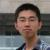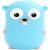0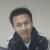...随便就来提问了，自己终结它，刚才彻底的看了下源代码（这是个好东西）

HashSet的contain方法代码：

```map = new HashMap<>(initialCapacity);

public boolean contains(Object o) {
return map.containsKey(o);
}```

```public boolean contains(Object o) {
return containsKey(o);
}

public boolean containsKey(Object key) {
return getEntry(key) != null;
}     final Entry<K,V> getEntry(Object key) {
int hash = (key == null) ? 0 : hash(key.hashCode());
for (Entry<K,V> e = table[indexFor(hash, table.length)];
e != null;
e = e.next) {
Object k;
if (e.hash == hash &&
((k = e.key) == key || (key != null && key.equals(k))))
return e;
}
return null;
}```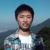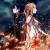0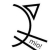0```package test;

import java.util.Arrays;
import java.util.BitSet;

public class TestIntSearch {
public static void main(String[] args) {
int[] array = { 1, 2, 2, 3, 4, 4, 5 };
BitSet bitSet = toBitSet(array);

System.out.println(binarySearch(array, 2));
System.out.println(binarySearch(array, 6));
System.out.println(bitSetSearch(bitSet, 2));
System.out.println(bitSetSearch(bitSet, 6));
}

public static BitSet toBitSet(int[] array) {
BitSet bitSet = new BitSet();
for (int e : array) {
bitSet.set(e);
}
return bitSet;
}

public static boolean binarySearch(int[] array, int value) {
return Arrays.binarySearch(array, value) >= 0;
}

public static boolean bitSetSearch(BitSet bitSet, int value) {
return bitSet.get(value);
}
}```
00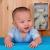0```package test;

import java.util.Arrays;

public class FindMaxNumber {
public static void main(String[] args) {
int[] array = randomArray(100);

// sort
Arrays.sort(array);

// find max number
boolean found = false;
for (int x = array.length - 1; x >= 0; --x) {
int ax = array[x];

for (int t = x - 1; t >= 0; --t) {
int at = array[t];
int ai = ax - at;
if (Arrays.binarySearch(array, 0, x, ai) >= 0) {
System.out.println("a[i]:" + ai);
System.out.println("a[t]:" + at);
System.out.println("a[x]:" + ax);
found = true;
break;
}
}

if (found) {
break;
}
}
}

private static int[] randomArray(int n) {
int[] array = new int[n];
for (int i = 0; i < n; i++) {
array[i] = (int) (Math.random() * i * 100) + 1;
}
return array;
}
}```
0